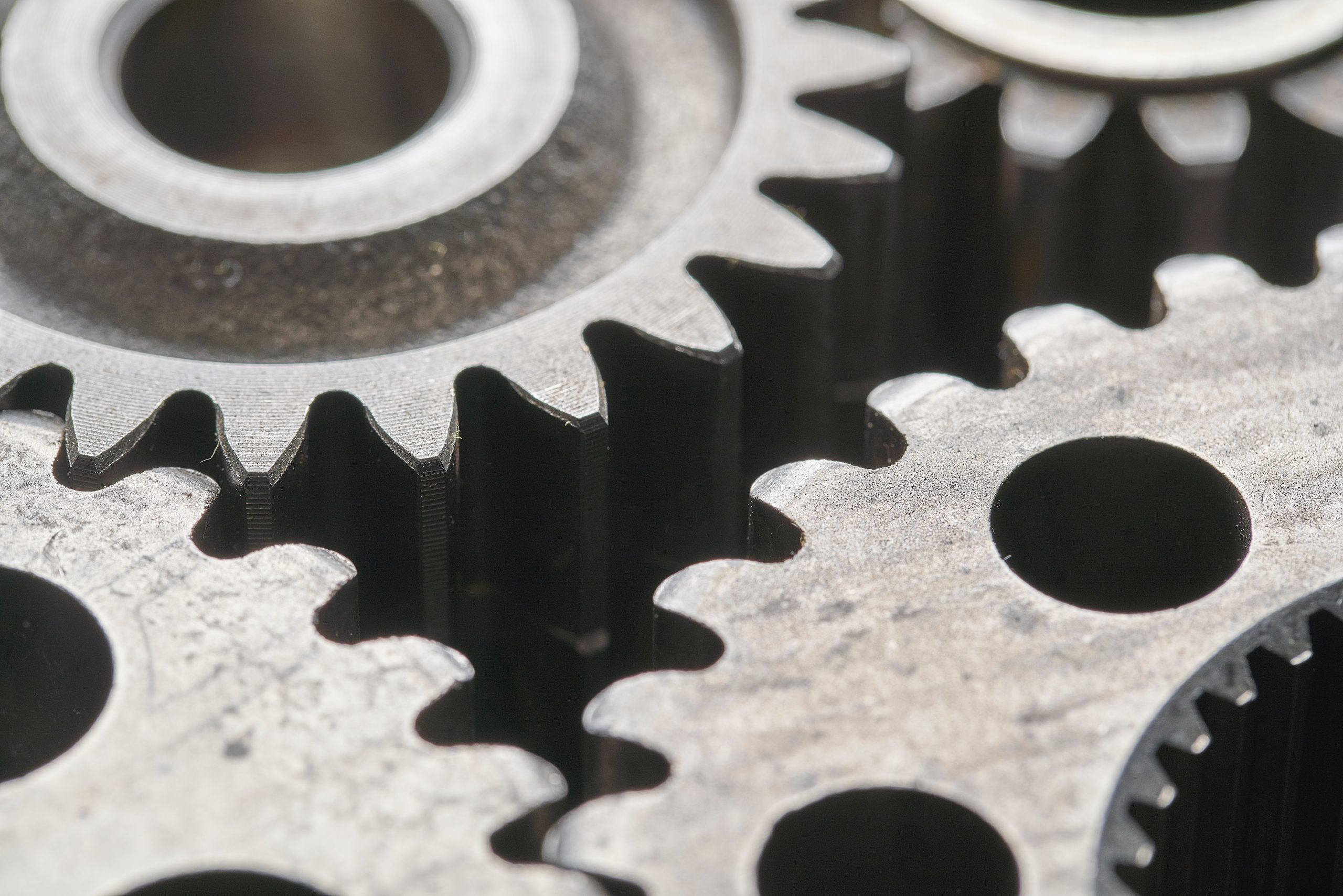# Fuel Consumption in Neutral Gear

· Timo Denk

When a car is in neutral gear it still consumes fuel. The reason is that it has to keep the engine running which means keeping it above the engine’s specific min. revolutions per minute (rpm). For my testing car, a Toyota Yaris XP 90 (second generation; 3 doors; 1.33 liter 4 cylinder engine) this value equals approximately 600 rpm.

In order to determine the fuel flow in neutral gear I switched into neutral while driving at 80 km/h and checked the predicted fuel consumption for 100 km. The display showed 0.8l per 100km. The formula to calculate the volume flow $\dot V$ from volume $V$, speed $s$, and velocity $v$ is the following:
$$\dot V=\frac{V}{\frac{s}{v}}=\frac{0.8l}{\frac{100km}{80\frac{km}{h}}}=0.64\frac{l}{h}$$